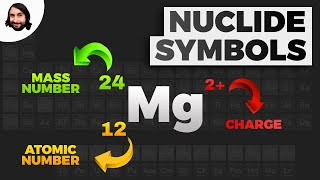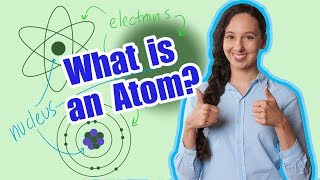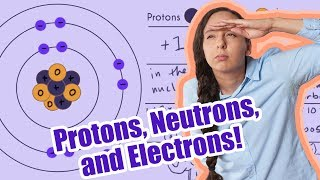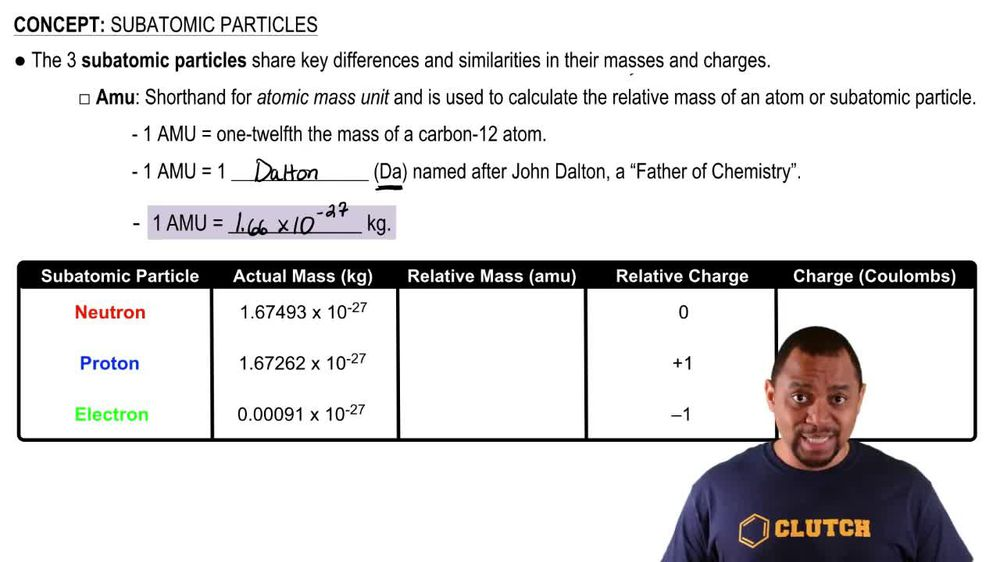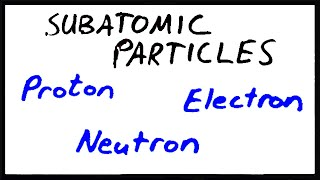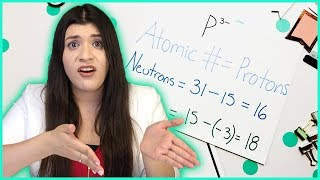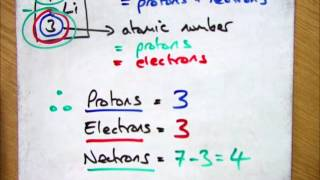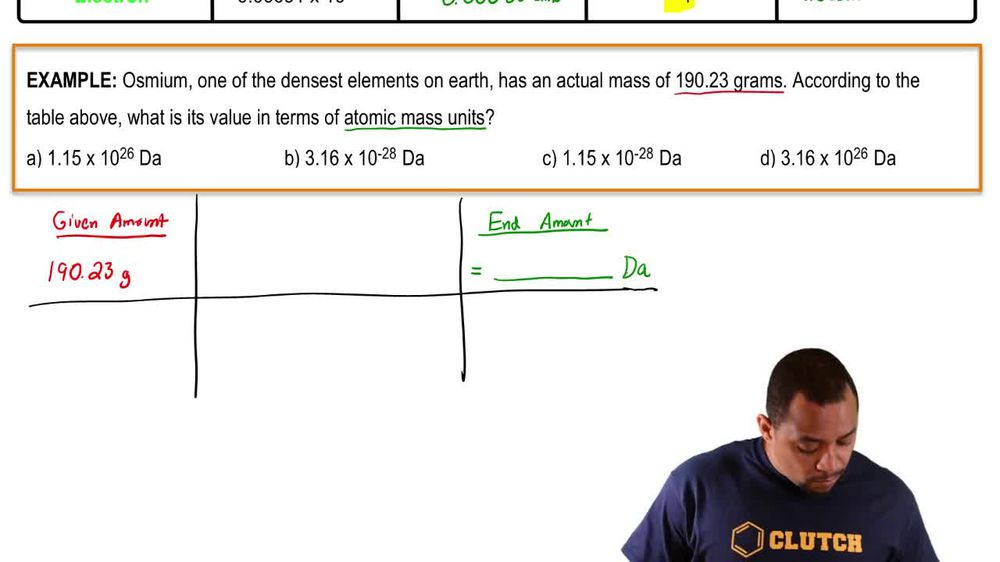Start typing, then use the up and down arrows to select an option from the list.
1. 2. Atoms & Elements2. Subatomic Particles
Problem

# Fill in the gaps in the following table, assuming each column represents a neutral atom. Symbol 159Tb Protons 29 37 Neutrons 34 53 Electrons 42 34 Mass no. 79 85 Complete the second row of the table excluding the row with isotope symbol.

Relevant Solution1m
Play a video:
Hi everyone here we have a question asking us to consider the following table showing symbols, the number of protons, electrons and neutrons and the mass number. Each column represents a neutral atom and our goal is to fill in the third column of this table. So one key aspect here is that it represents a neutral atom, which means the number of electrons is going to equal the number of protons. So we know we have 50 electrons, which means we will also have 50 protons. And our protons is the same as our atomic number. So if we look on the periodic table for the atomic number 50, we see that it is 10. And to find the mass number, the mass number equals the number of protons plus the number of neutrons, Which in this case is plus 50 Which equals 118. So our mass number is 118 And we will have a 118 above the 10 symbol. So that is our final answer. Thank you for watching. Bye.